Courses

# Which of the following is not an empty set?a){x : x is a multiple of 7, x < 7, x ∈ N}b)Set of common points of two parallel lines in a planec){x : 6 + 2x > 5x + 3, x ∈ N}d)Set of smallest whole numberCorrect answer is 'D'. Can you explain this answer?

## JEE Question

By Abhishek Dubey · Dec 12, 2019 ·JEE
Shruti Dubey answered Dec 09, 2019
D

Anand Kumar answered Feb 15, 2020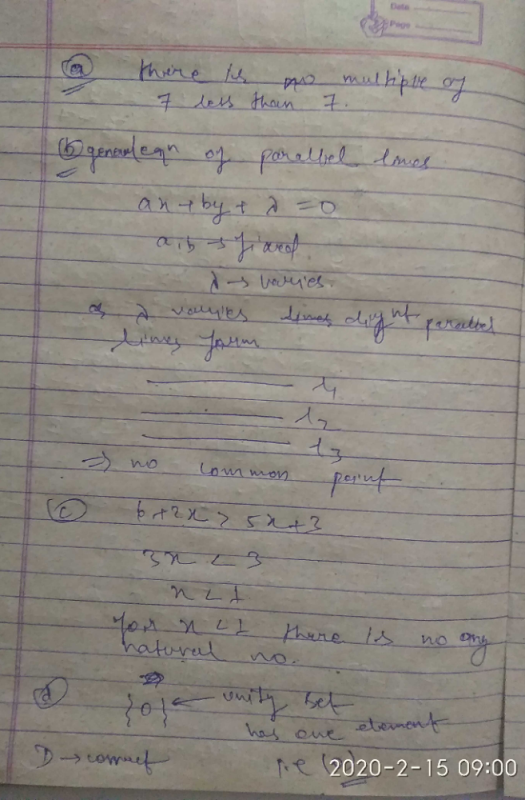Hitesh Kumar answered Mar 25, 2020
A

Shalini Nawariya answered Mar 25, 2020
Yes D will be the right answer because in all the statement , set will not consist any element satisfying given condition except option D as 0 is the smallest whole number as per given .

Khaja Moinuddin answered May 03, 2020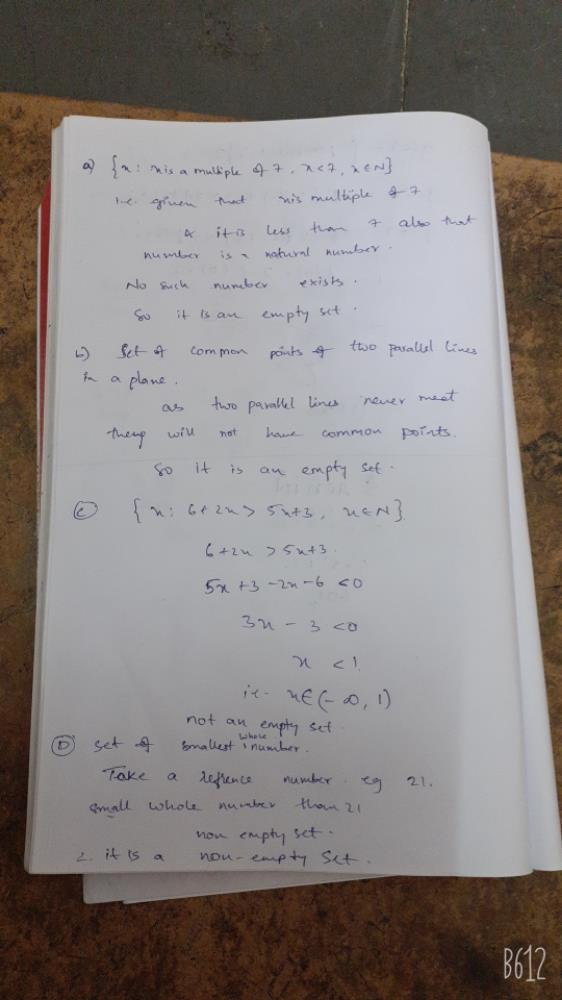Mysterio Man answered Jul 12, 2020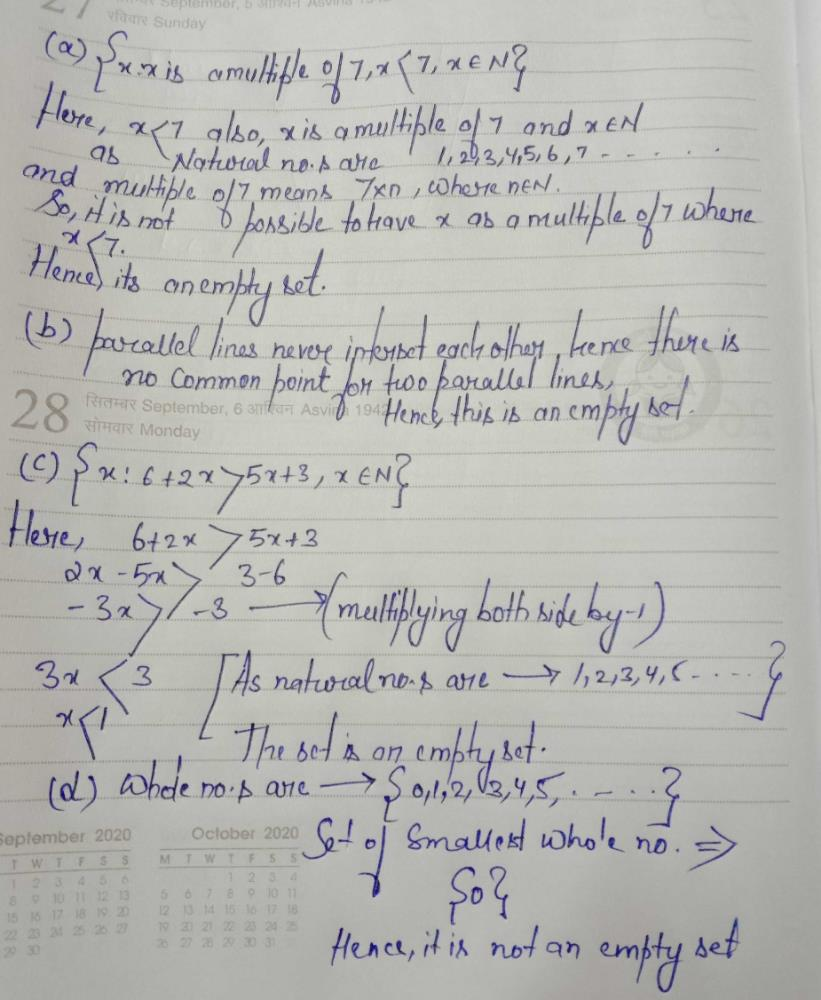Shashmith Shashmith answered Mar 24, 2020

Shweta Singh answered May 03, 2020
It is not defined that how many numbers are smallest whole number as it is given in question to write the set of smallest whole number.

Uppuluri Sv Subbarao answered May 02, 2020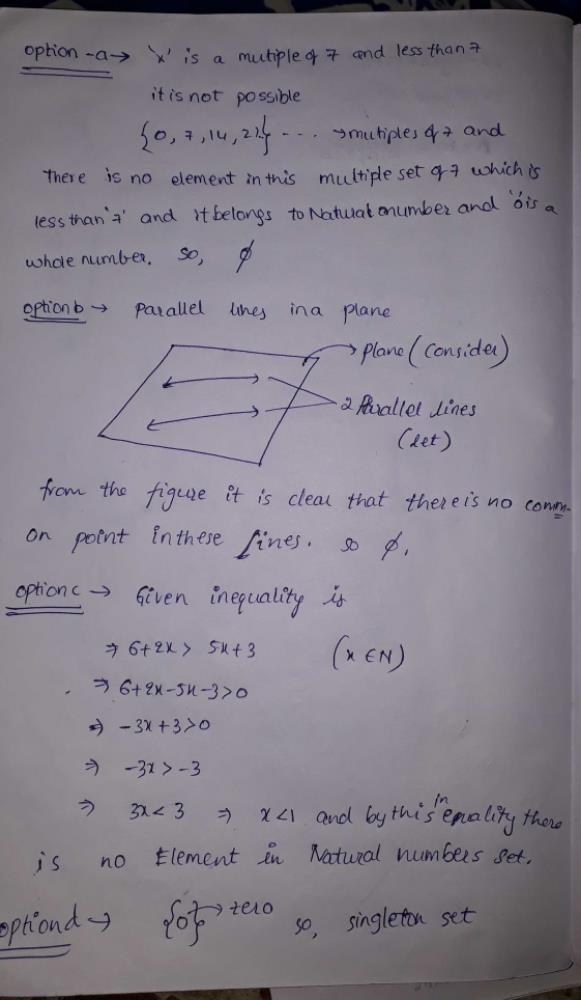Nishant Sharma answered May 19, 2020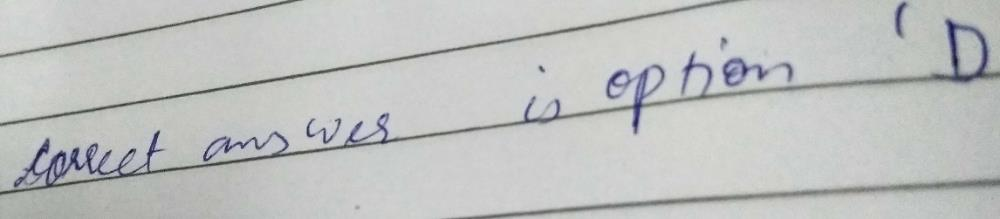Yashwanth Kota answered Jul 29, 2020
Option D is correct because in D "0" is an element so it's not an empty set

Anupam Jain answered Apr 18, 2020
Yes ,buddy correct ans is 'D' Because it have element {0}
so it is correct

Shashank Jaiswal answered May 26, 2018
As, the set of Whole numbers is {0, 1, 2, 3, 4, 5, 6, 7, 8, 9, ...};
So, according to the set of whole numbers above, the smallest whole number should be "0" and therefore the set of smallest whole number is not empty.

Tarun Mahawar answered Apr 15, 2020
Set of smallest whole number is {0}, which is a non empty set.So, correct answer is option is (D).

Roshni Mohapatra answered Sep 13, 2020
As because no other options are valid for sets containing elements...option D is the correct option because this set contains the element 0(the smallest whole number).

Rajendra Kumar answered Jun 01, 2020
B

Kamali Anandbabu answered Sep 10, 2020
Option D is correct.
If u see in option A, multiples of 7 is 7,14, 21, so on.. It is given that x is less than 7, therefore option A is an empty set.
In option B, there is no common points between two parallel lines in a plane because that two lines do not intersect. So this option is also an empty set.
In option C, x should be in negative then only this condition will be satisfied because for example if we put 1 for x it will come like this 8>8, this is not be satisfied. If we put -1 for x it will 4>-2. This can be satisfied but it is given that x belongs to natural number. Therefore this option is also an empty set.
In option D, the set is ( 0, 1, 2, 3, so on..) according to the condition, the set of smallest whole number is (0). Therefore option D is not an empty set.

Peter Parker answered Apr 03, 2020
Option D is correct.

Girish Shukla answered Feb 18, 2020

Nisha Sharma answered May 22, 2020
Answer is D( Set of smaller whole number)

Sanghamitra Maithani answered Sep 24, 2020
D as rest are empty sets

Aman Choudhary answered Jul 23, 2020
Because the smallest number set is not actually it is an imznary number called negative infinite

Sakshi Dombe answered Sep 22, 2020
D is the right answer cause here is not given any condition or range cor whole numbers.

Amrit Raj Garg answered Aug 04, 2020
I m felling sleepy so I won't answer

Raveendra Chandagani answered May 29, 2020
O is small est whole no

Anjali Sharma answered Aug 14, 2020

Lahari Mothukuri answered Jun 01, 2020
If you see option( a ) x can be 7,14,21and so on but according to given condition these numbers are not less than 7 hence it is an empty set

if you see option (b) two parallel lines cannot intersect each other so there is no common point hence it is an empty set

if you see otion (c) 6+2x>5x+3 can only be possible when x is a negative number but according to given condition x should be natural number hence it is an empty set

if you see option (d) whole numbers are {0,1,2,3,4,and so on} in this smallest whole number is {0} hence it is not an empty set. can also say it as singleton set

D option is correct
the reason is smallest whole number is zero
and zero and fie are different things fie represents null set whereas zero may or may not be element of any sets
hence in this case the answer is {0}
therefore it's not empty set
hope you guys understood it :-)

Netika Sharma answered Jun 25, 2020
Option d

Vandana Gupta answered Mar 23, 2020
Smallest whole number cannot make a set because it is single term and single terms do not make sets.

Nishtha Hari answered Jun 13, 2020
In option (a) we write it in roster form;
{ } or phi .....phi is member of every set so it will come in every set.
in option (b) we write it in roster form;
{ } or phi as there is no common point between two parallel lines, they can never intersect.
in option (c) in roster form;
{ } or phi....if we take natural no. 1
6+2(1)=8. 5(1)+3=8
as 8 cannot be big no. than itself so ans. is phi
but in opt (d);
{0}, phi .....as phi is member of every set so there is phi as well as 1 member so it is not considered as a null set hence opt (d) is correct one.

Bhargav Sompura answered Jun 26, 2020
D is correct option as it has one element  in it and all other are empty set.

Because smallest whole number is 0 and that is a null set

Ayomide Attah answered Aug 31, 2020
The smallest whole number is 1, so it is not an empty set

Kurakula Suryateja answered Aug 19, 2020
(D) is the correct answer because smallest whole number is 0 if it is a set it would be like this {0}
if any element in a set it would be a non empty set
so option d is correct

Dr Stephen Strange answered Jan 07, 2020
because
multiple of 7 can't be smaller than 7 itself.

Ankit Singh answered Feb 03, 2020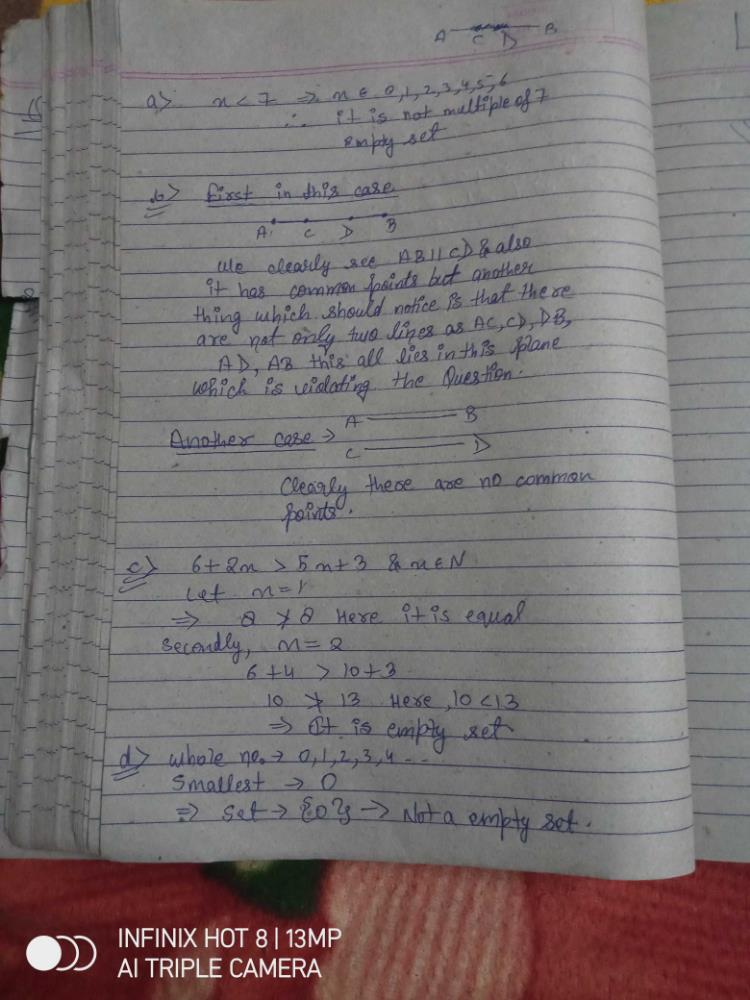Shivam Kumar answered Jun 22, 2020
0,1,2,3,4...are comes whole number ,
so it cannot be a null set

Prateek Raj answered Feb 13, 2020
Correct answer is d because option a, b, c, are empty set.
(A) - In table of 7 any number is not less than 7 when x belongs to natural number. ex:- 7,14,21,28,35,42,49,56,etc thus, (empty set)

(B) - In these parallel lines: == or I I . There were no intersection points or no common points as you see. ( distance between two or more parallel lines remain always equal) . thus, (empty set)

(C) - as you put the values of x, where x is a natural number
then you get the same value for x=1 but for greater than 1
you get the value of 5x+3 more than 6+2x which is not satisfying by the given condition. thus, (empty set)

(D) - whole numbers : 0,1,2,3,4,5,6,7,8,9,10,11,etc
set of smallest whole number is 0 - {0}.thus,(Singleton set)
not an empty set.

Manash Hial answered Aug 22, 2020
If one knows basic maths too he can easily say first three options are empty set coz no elements fits the conditions. About ans D the smallest whole no is 0 ,so the set is not empty that it is a singleton set with one element 0.

Kartik Swami answered Jan 07, 2020
Smallest whole number is zero which can't be a empty set

Shaurya Sawai answered Sep 15, 2020
Correct answer is d as smallest whole number is 0 so {0} is not a empty set as it contain one element which is 0.

K Sankara Rao answered 2 weeks ago
Option A is an empty set because there would be no multiple of 7 which is less than 7 and belonging to the set of natural numbers . option b is also an empty set because there would be no common points to 2 parallel lines . option c is also an empty set because if you choose any random number the given statement will also be wrong there fore it is also an empty set.and the last option d the smallest whole number is 0, it is not an empty set ,so d is the right answer

Ravi Teja answered Mar 31, 2020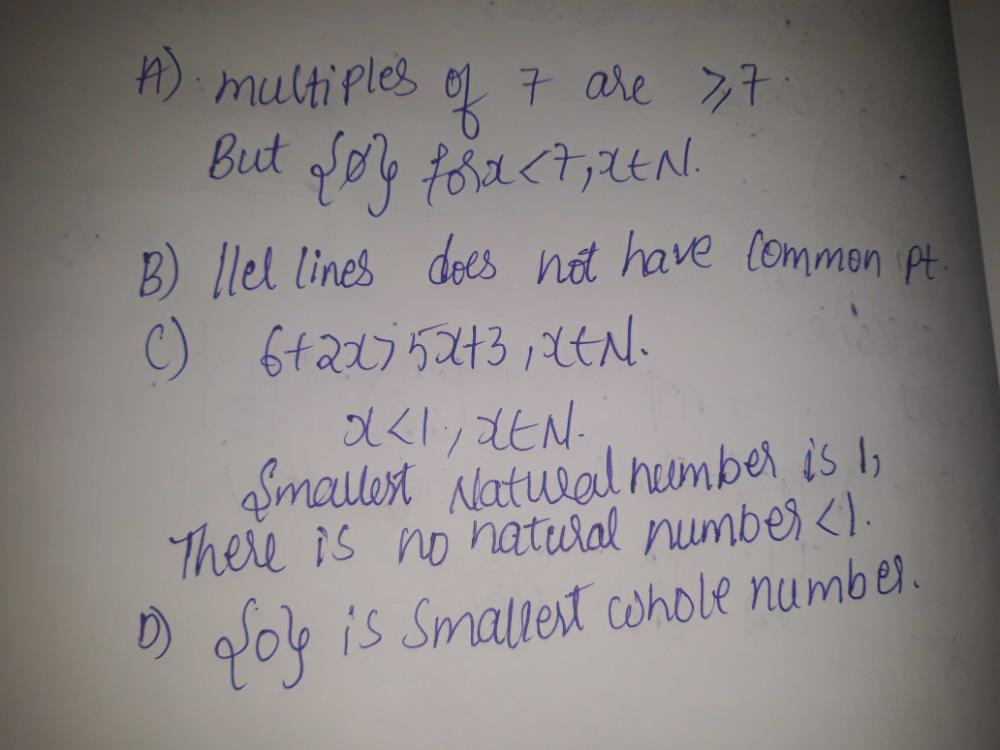Anjali Malviya answered Jul 08, 2020
Yess
set of smallest whole number = { 0 }
which is not an empty set
no. of elements in above set is 1

Piyush Sharma answered Sep 13, 2020
D is the correct option bcoz set of smallest whole no is {0}

Shrawankumar Shrawankuma answered Jun 23, 2020
Goood

Sahil Rana answered Jan 08, 2020

Raja Kotni answered Jul 16, 2020
Option d is the correct because remaining options are empty sets

Shasatran Rudra answered Apr 07, 2020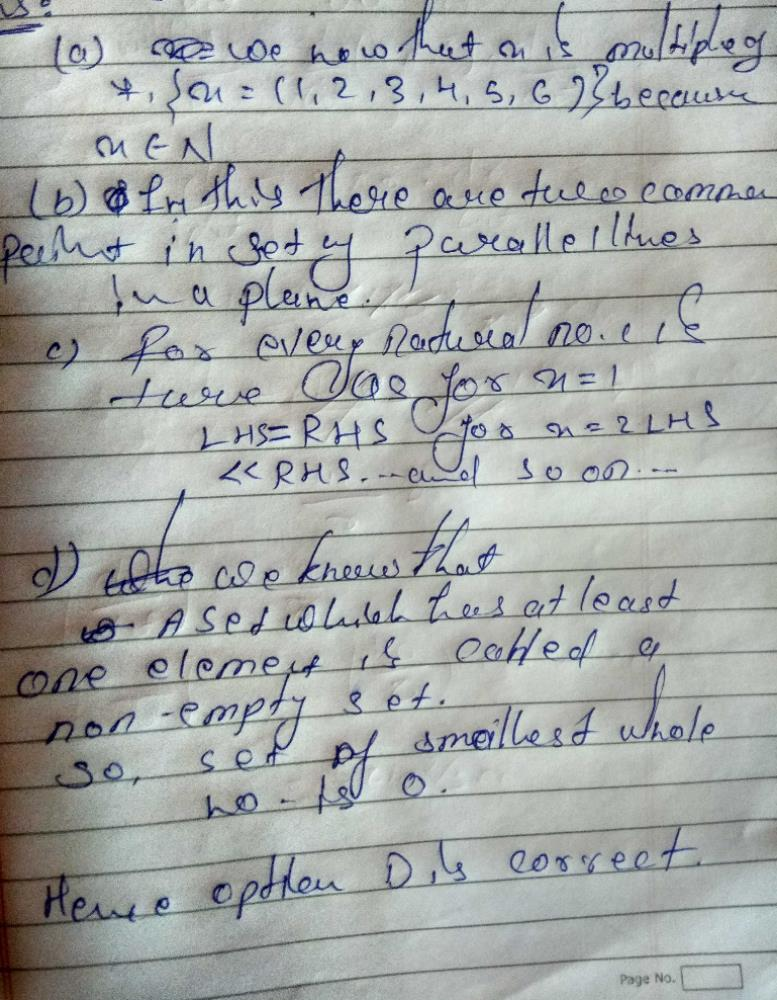Vivek Sagarwal answered Apr 08, 2020
Set of smallest number is start from 0

Shikha Singh Shikha Sing answered Jul 07, 2020
D is a correct option

Tanisha Kalia answered Jun 14, 2020

Abhitej Tiwari answered Sep 13, 2020
Surely many find that first 2 options are a void set and 4th consist of { '0' } only
for 3 we can prove it as follows
given -
6+2x > 5x+3 which means
2x > 5x-3 (subtract 3 from both sides) and
x > 5x-3/2
it can be easily seen that this property doesn't hold's good.
and so the set is a null set !!

note: at x= 1 in the given eq.
1is not greater than 1.
it is equal to it.

Waseem Raja Wasi answered Apr 03, 2020
0 is a samalest whole number . therefore it's not empty set {0}

Devansh Raj answered May 31, 2020

Sarika Bharti answered May 01, 2020
Correct option is D because it has an element 0 while in all other options a null set became.

Diya Bhardwaj answered May 11, 2020
D option is correct

Anjali Kumari answered May 27, 2020
It is because smallest whole number is 0 which is null or empty set

Saravanan J answered Jun 30, 2020

Rohit Kumar answered Sep 19, 2020
And: (d), as smallest whole number is zero and so it's set contain it's element as zero.

Shreya Chauhan answered Aug 29, 2020
Correct ans is D

Chinmay Mahajan answered Mar 23, 2020
Set of smallest whole number can't be defined because smallest whole number is 0 and so to which limits the number are involved can't be explained hence d is the correct explanation

Sagar Pandey answered Jan 23, 2020
Option 1,2and3clearly an empty set but option d is not an empty set because it contains element:
w={0,1,2,3,4,5,6,7...}

Abhi Gupta answered May 21, 2020

Rahul Kumar answered Jun 10, 2020
(a) x € fi
(b) empty set
(c) 6 + 2x > 5x + 3
or,. 3 > 3x
or ,
x < />
now,
x € fi
(d) if we see 1 is the smallest whole number for 2 , 3 etc similar for all thus it's set = {0 , 1 , 2 , 3 , .....}
which is not empty set.
hence d is the correct because

Saurabh Rajput answered Aug 14, 2020
D is correct answer . it is a set of well defined object because smallest whole number is 0

Mani Chari answered May 28, 2020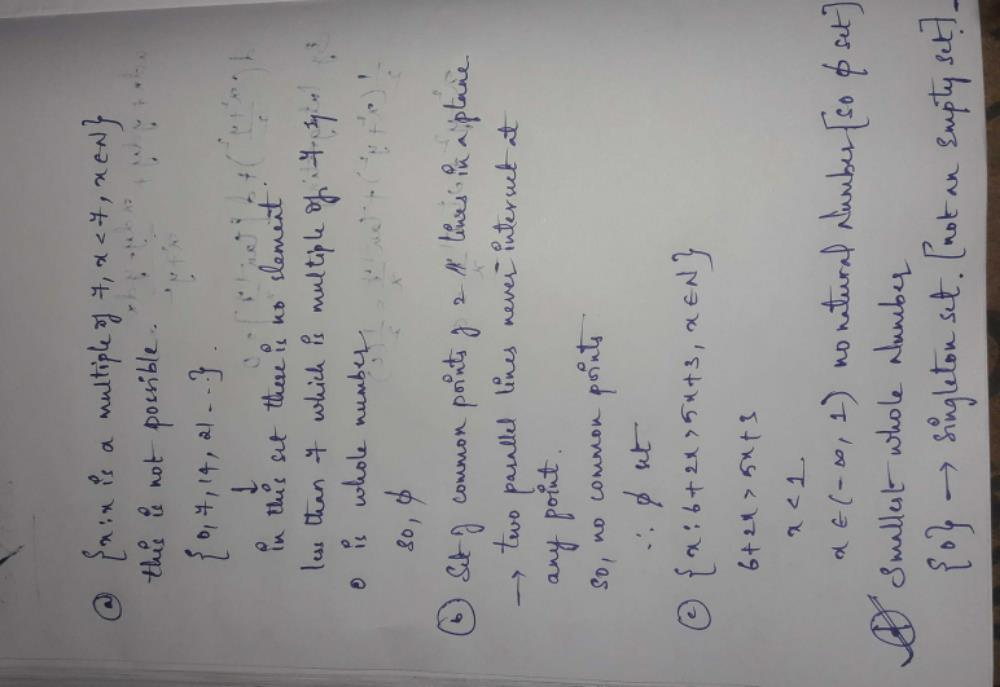Anilkumar Bharti answered Jun 05, 2020
Set of smallest whole no conatains 0 as its element...

option c could have been a non empty set until it's an integer and it is mentioned natural numbers...so an empty set
option b ....two parallel lines have no solution so an empty set for common point...
similarly in option a x cannot be the multiple of 7 until it's negative and integers are not allowed so also an empty set

Asiya Anjum answered Jun 01, 2020
Set of smallest whole number is 0.,so it is not an empty set.....
hence option D is correct

Vikas Singh answered 3 weeks ago

Anita Gupta answered Jan 03, 2020
D is right because there is only 1 smallest whole no. so it can't be empty set

Vansh Bhardwaj answered Dec 12, 2019
D- Set of smallest whole no. which has definite value

Shibani Das answered Jul 12, 2020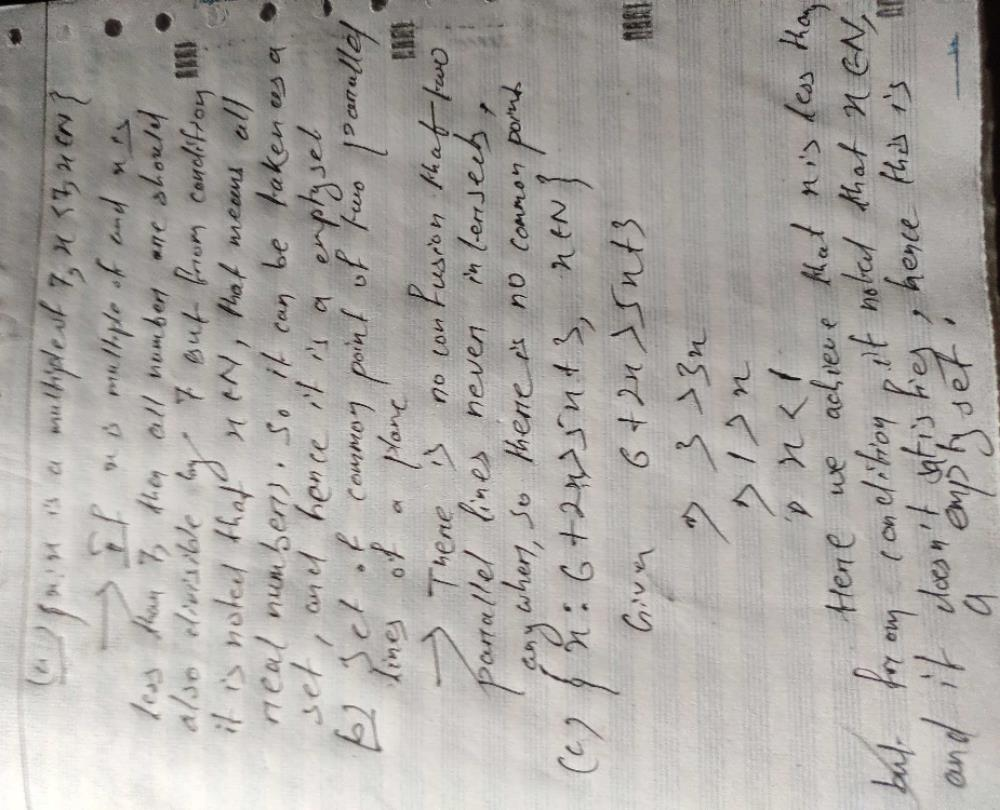Varshitha Bittu answered Jun 18, 2020
D
x<7=0 but="" not="" natural="" number="" so="" empty="" set="">
2.parallel lines never coincide so empty set
3.on solving we get x<1=0 but="" does="" not="" belongs="" to="" natural="" number="" so="" empty="" set="">
4.smallest whole number is 0 so it is not a empty set

Khirod Mondal answered Jun 04, 2020
Yes D option is right because one element is exist in this set . 0 is the smallest whole number

Avula Vivekanandam answered Apr 20, 2020
Yes D option is right, because in above three options are null set, fourth option is not null set because smallest whole number is 0 (zero)

Rajendra Singh Bhandari answered May 20, 2020
All option contain no elements in there sets
but option D contain a number 0 in its set so D should be correct ans

Stuti Kumari answered Jun 09, 2020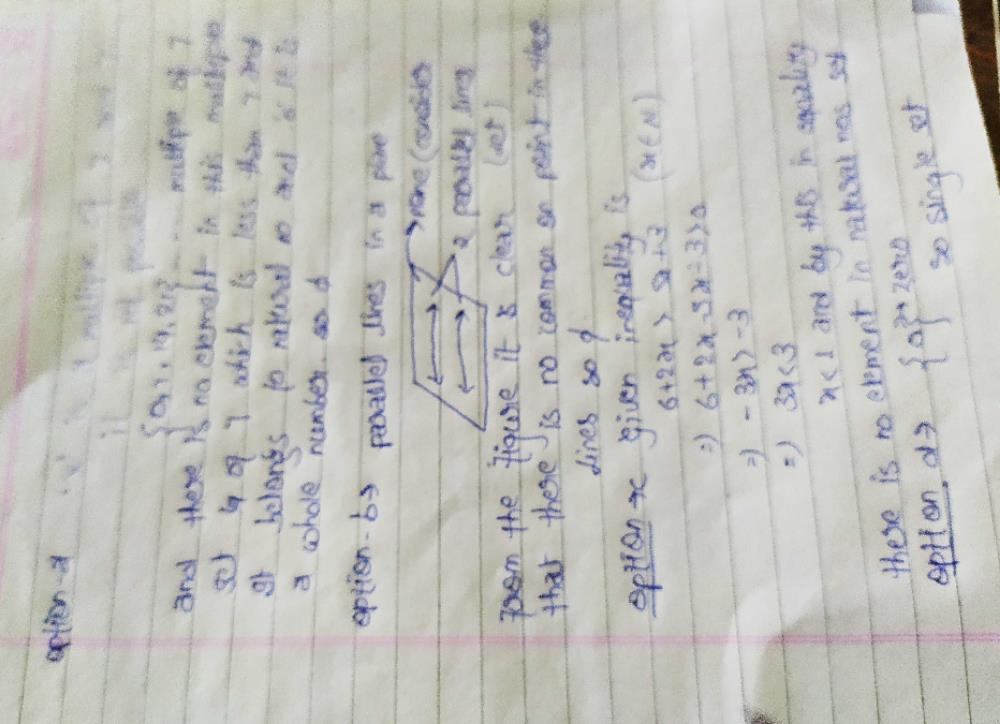Ananta Kumari answered Jul 04, 2020
Smallest whole number is 0,which is count as one element of the set

Ajinkya Kutarmare answered Jul 20, 2020

Shashank Shekhar answered Jul 26, 2020
D because
smallest whole number is is zero so the set will exist

Abbrar .m answered Jul 30, 2020
Yes d is the correct answer.

Bharti Gupta answered Aug 12, 2020
D ans is correct bcoz it has {0} in its set

Dazle Roger answered Aug 17, 2020
As you that 0 is the smallest whole number and 0 is a empty set so d 8s correct

Jayant Kumar answered Jul 29, 2020
D is correct , because it is smallest whole number and it's set is possible as { { },{0} }. And rest all are empty set.

Harsh Kumar answered Sep 11, 2020
(D ) because in this set there is an element 0